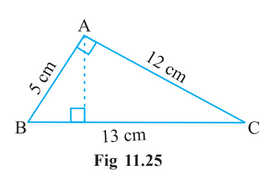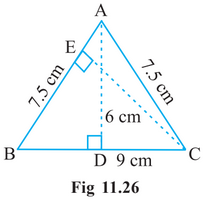Q

# ∆ABC is right angled at A (Fig 11.25). AD is perpendicular to BC. If AB = 5 cm, BC = 13 cm and AC = 12 cm, Find the area of ∆ABC. Also find the length of AD.

Q. 7.    is right angled at   (Fig 11.25). is perpendicular to .  If and Find the area of

. Also find the length of.Views
R

We know that

Area of triangle

Now,

When base = 5 cm and height = 12 cm

Then, the area is equal to

Now,

When base = 13 cm and height = AD area remain same

Therefore,

Therefore, value of AD is    and the area is equal to

Exams
Articles
Questions